# Isosceles Trapezoid

## Isosceles trapezoid definition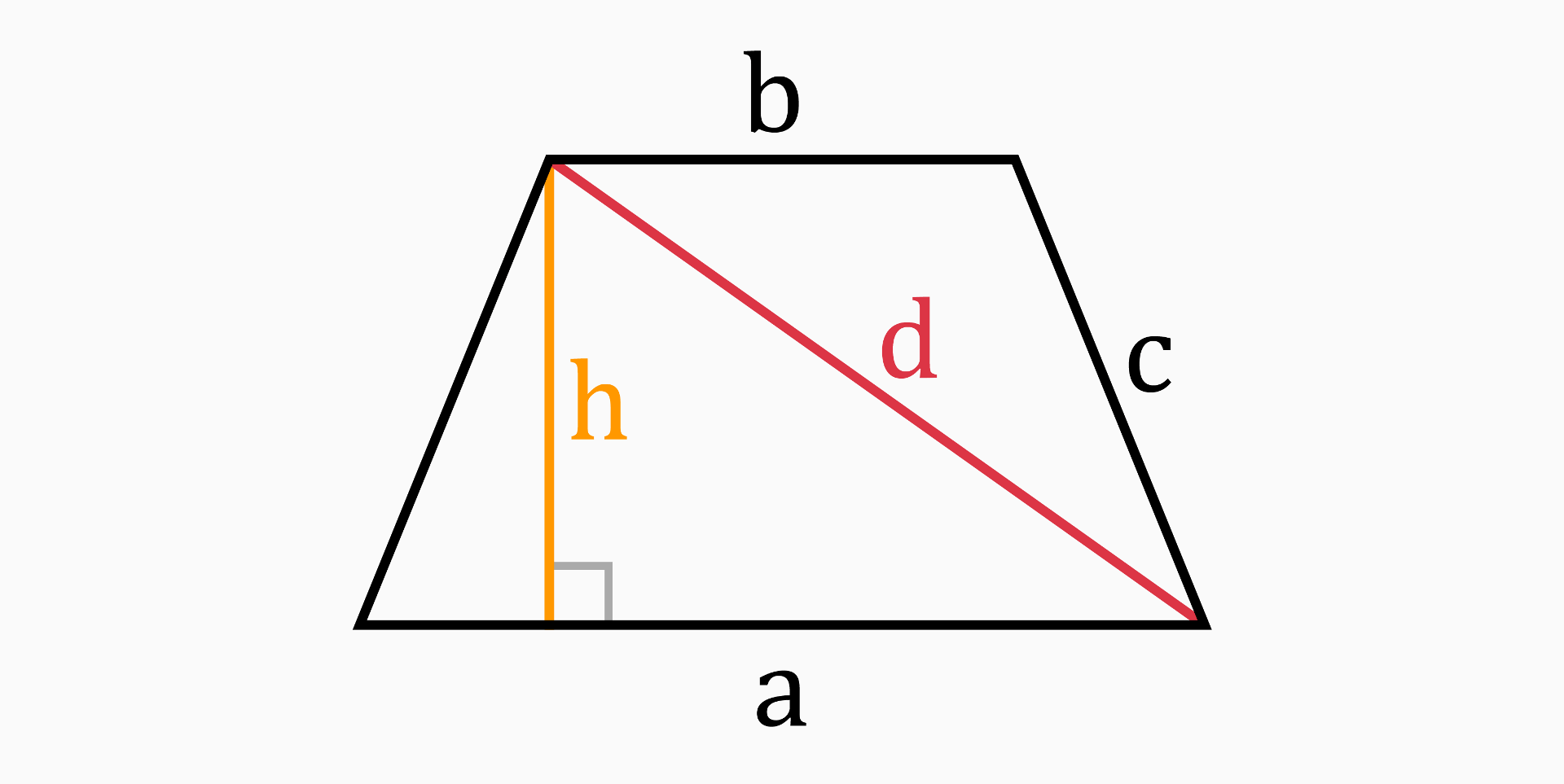An isosceles trapezoid (or trapez) is a trapezoid with legs that have equal length.

A trapezoid is a geometric shape with four sides, where at least one pair of sides is parallel to each other. The two parallel sides are called bases, and the other two sides are called legs. If the legs are also parallel to each other, then we get a parallelogram.

## Parts of an isosceles trapezoid

There are eight parts of an isosceles trapezoid mentioned in this tool: long side (a), short side (b), legs (c), height (h), area (A), perimeter (P), diagonal (d) and circumradius (R).

### Long and short sides (a, b)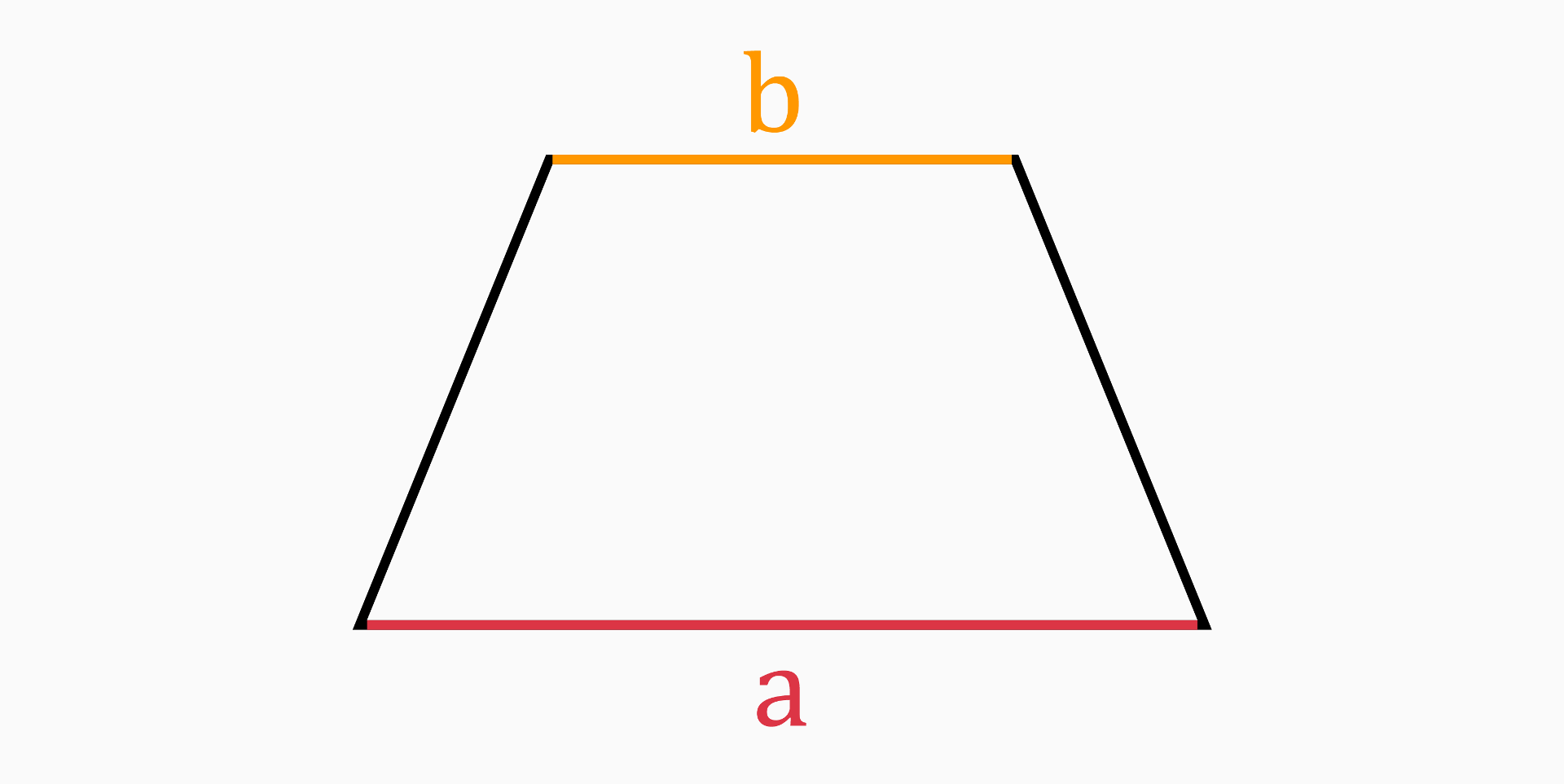The long and short sides are the two sides that are parallel to each other. These two sides are known as the bases of the isosceles trapezoid.

In our case, the long side (a) is the bottom base and the short side (b) is the top base of the trapezoid.

To be able to calculate all values of an isosceles trapezoid, you must know the value of at least one of the base sides. You can calculate the second base with one of the following formulas:

To calculate all the values of an isosceles trapezoid, you need to know the value of at least one of the base sides and two other values. Then you can calculate the second base with one of the following formulas:

• If you know the area and height:

`a = 2 * A / h - b`

`b = 2 * A / h - a`

• If you know the height and diagonal:

`a = 2 * √(d² - h²) - b`

`b = 2 * √(d² - h²) - a`

• If you know the diagonal and leg length:

`a = (d² - c²) / b`

`b = (d² - c²) / a`

• If you know the leg length and perimeter:

`a = P - b - 2 * c`

`b = P - a - 2 * c`

### Legs (c)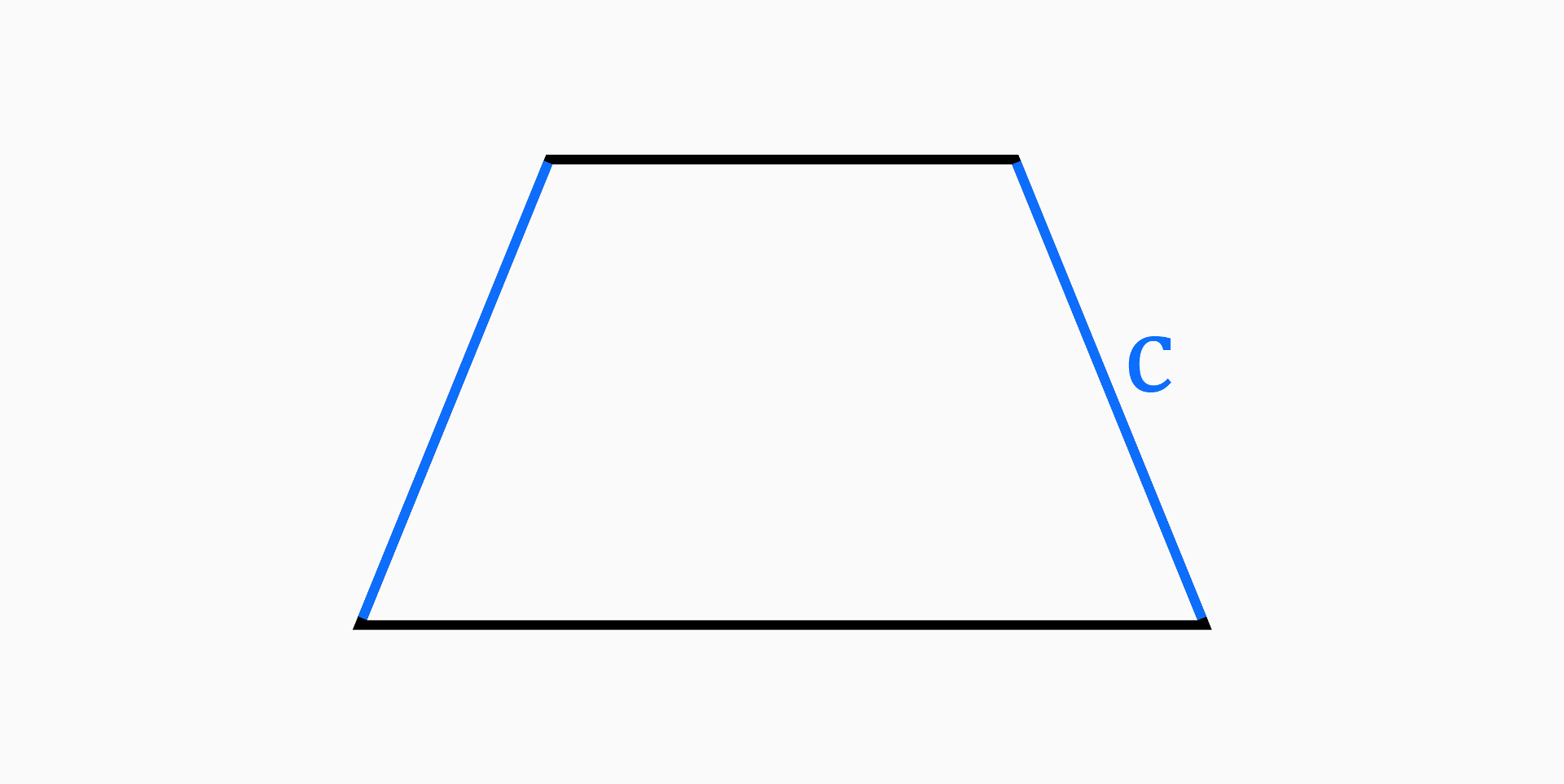The legs of an isosceles trapezoid are the two sides that are equal in length and connect the parallel sides (bases). They also form two equal angles at the end of each parallel side.

To calculate the leg length, you need to know the length of the long base, the short base and one other value. Then you can use one of the following formulas:

• If you know the height:

`c = √(4 * h² + (a - b)²) / 2`

• If you know the diagonal:

`c = √(d² - a * b)`

• If you know the perimeter:

`c = (P - a - b) / 2`

### Height (h)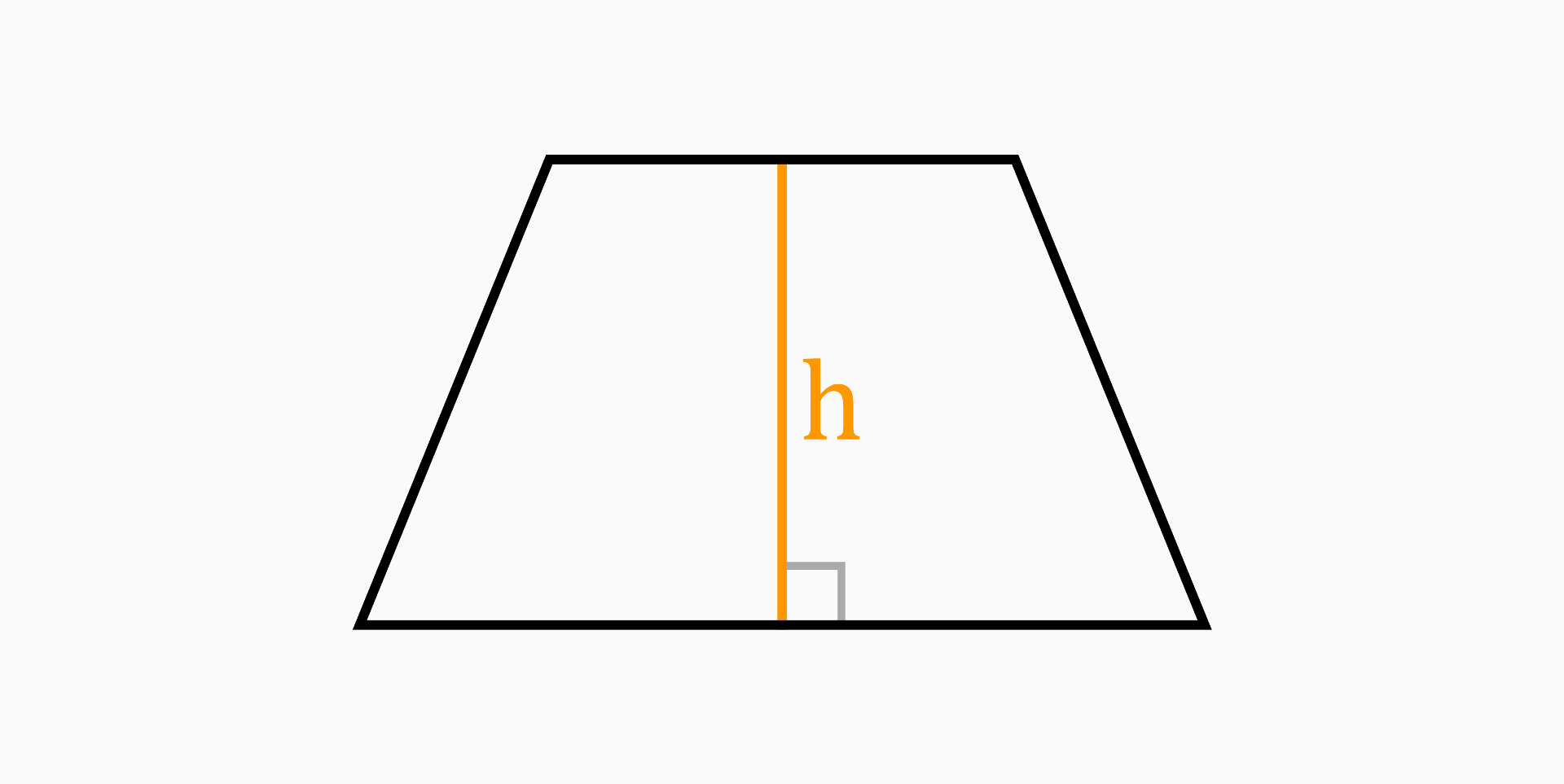The height of an isosceles trapezoid is the perpendicular distance between the two parallel sides.

It can be calculated with the following formulas:

• If you know all sides of the isosceles trapezoid:

`h = √(4 * c² - (a - b)²) / 2`

• If you know the two bases and the circumference:

`h = √(R² - a² / 4) + √(R² - b² / 4)`

### Area (A)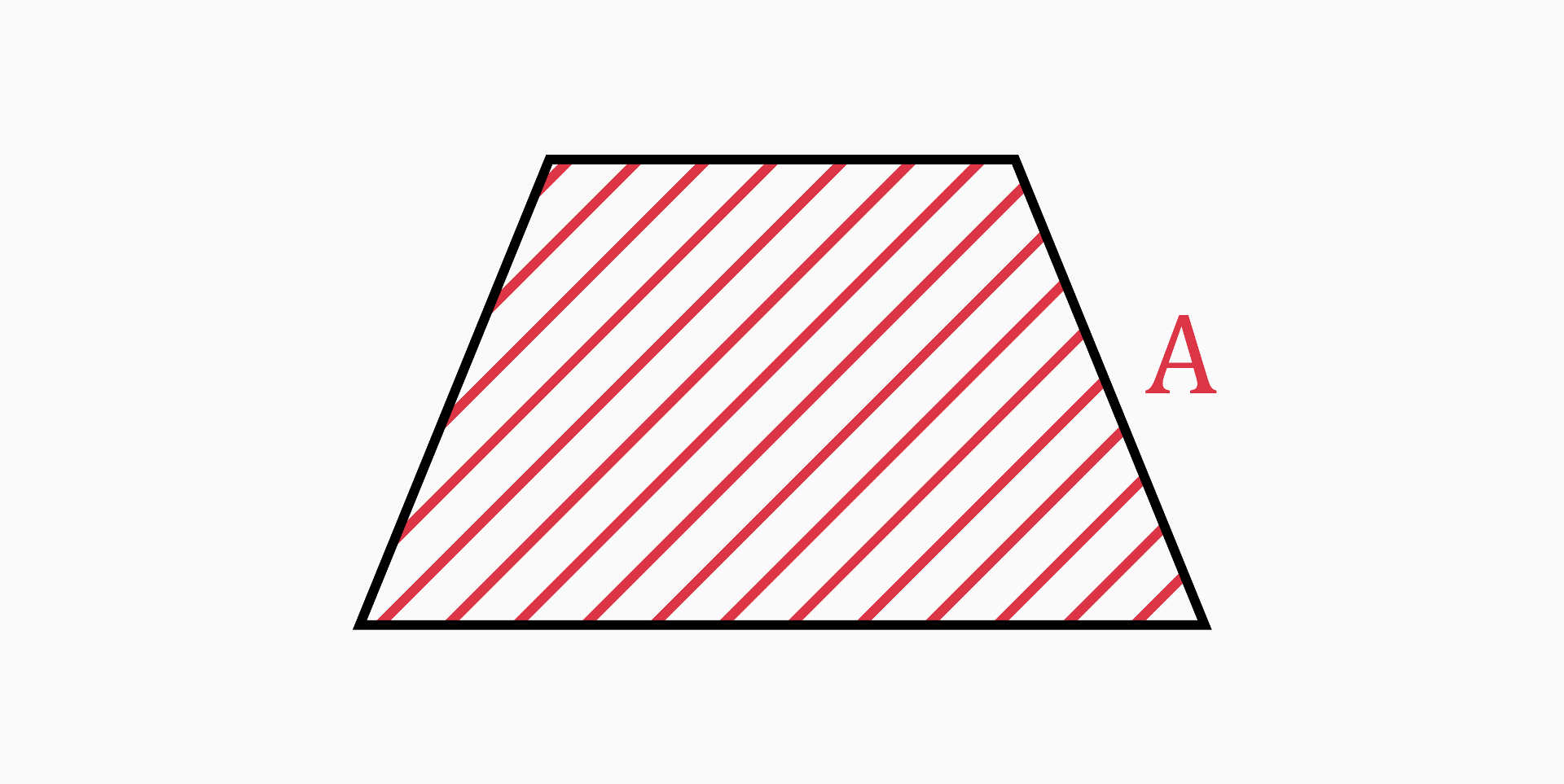The area of an isosceles trapezoid is the region enclosed inside the trapezoid.

It can be calculated by the formula:

`A = (a + b) / 2 * h`

### Perimeter (P)The perimeter of an isosceles trapezoid is the sum of the lengths of all its sides.

The formula for calculating the perimeter of an isosceles trapezoid is:

`P = a + b + 2 * c`

### Diagonal (d)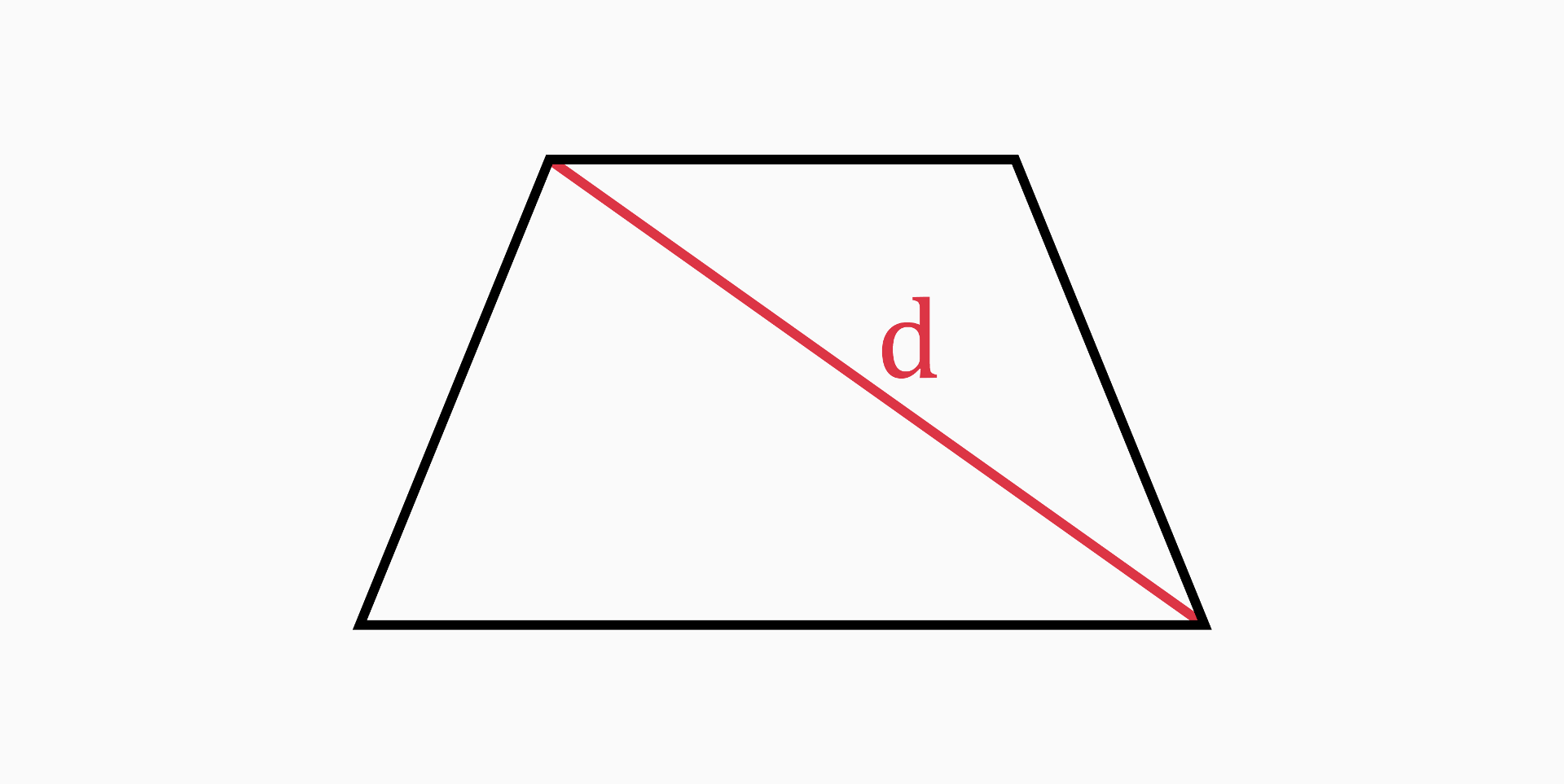A diagonal of an isosceles trapezoid is a line segment that connects two non-adjacent vertices. In every isosceles trapezoid there are two diagonals of equal length.

The formula for calculating the diagonal of an isosceles trapezoid is:

`d = √(a * b + c²)`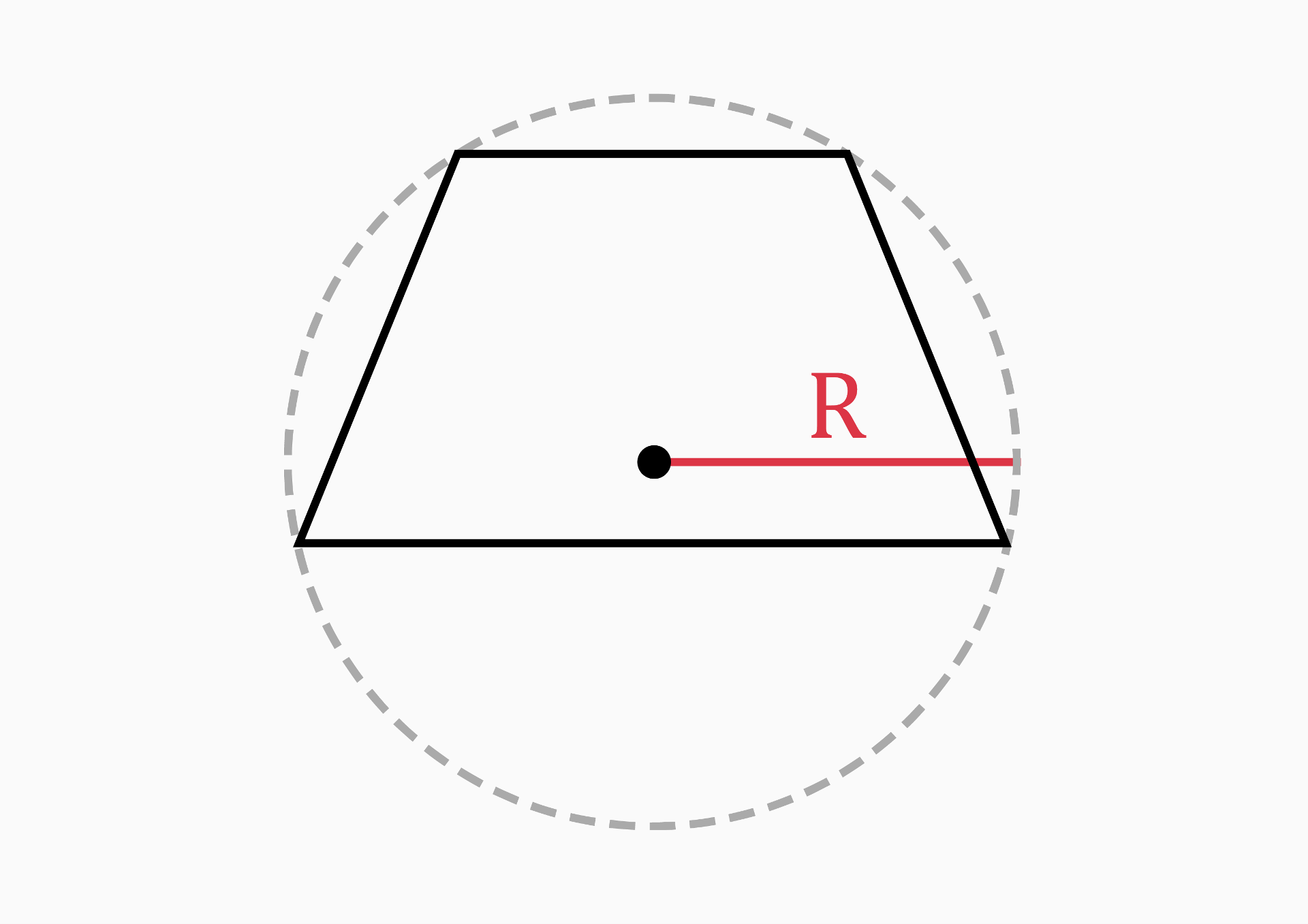``````R = (c*d*b) / 4 / √((c+d+b) / 2 * ((c+d+b) / 2 - c) * ((c+d+b) / 2 - d)*((c+d+b) / 2 - b))